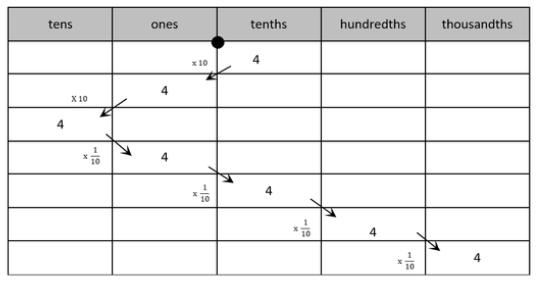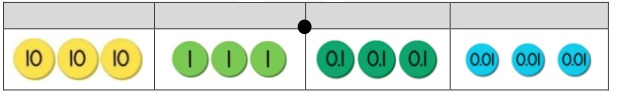# MA.5.NSO.1.1Export Print
Express how the value of a digit in a multi-digit number with decimals to the thousandths changes if the digit moves one or more places to the left or right.
General Information
Subject Area: Mathematics (B.E.S.T.)
Strand: Number Sense and Operations
Status: State Board Approved

## Benchmark Instructional Guide

• NA

### Vertical Alignment

Previous Benchmarks

Next Benchmarks

### Purpose and Instructional Strategies

This purpose of this benchmark is for students to reason about the magnitude of digits in a number. This benchmark extends the understanding from grade 4 (MA.4.NSO.1.1), where 1 represents 10 times what it represents in the place to its right and $\frac{\text{1}}{\text{10}}$ of what it represents in the students expressed their understanding that in multi-digit whole numbers, a digit in one place place to its left. All of this work forms the foundation for arithmetic and algorithms with decimals which is completed in grade 6 (MA.6.NSO.2.1).
• To help students understand the meaning of the 10 times and $\frac{\text{1}}{\text{10}}$ of relationship, students can use base ten manipulatives or simply bundle classroom objects (e.g., paper clips, pretzel sticks). Students should name numbers and use verbal descriptions to explain the relationship between numbers (e.g., “6 is 10 times greater than 6 tenths, and 6 tenths is $\frac{\text{1}}{\text{10}}$ of 6”). In addition to physical manipulatives, place value charts help students understand the relationship between digits in different places (MTR.2.1).
• Instruction of this benchmark should connect with student work with whole numbers. For example, students who understand 35 × 2 = 70 can reason that 3.5 × 2 = 7 because 3.5 is $\frac{\text{1}}{\text{10}}$ of 35, therefore its product with 2 will be $\frac{\text{1}}{\text{10}}$ of 70 (MTR.5.1).

### Common Misconceptions or Errors

• Students can misunderstand what “$\frac{\text{1}}{\text{10}}$of” a number represents. Teachers can connect of $\frac{\text{1}}{\text{10}}$ of to “ten times less” or “dividing by 10” to help students connect $\frac{\text{1}}{\text{10}}$ of a number to 10 times greater.
• Students who use either rule “move the decimal point” or “shift the digits” without understanding when multiplying by a power of ten can easily make errors. Students need to understand that from either point of view, the position of the decimal point marks the transition between the ones and the tenths place.

### Strategies to Support Tiered Instruction

• Instruction includes the use of place value charts and models such as place value disks to demonstrate how the value of a digit changes if the digit moves one place to the left or right. Explicit instruction includes using place value understanding to make the connections between the concepts of “$\frac{\text{1}}{\text{10}}$ of,” “ten times less” and “dividing by 10.” Place value charts are used to demonstrate that the decimal point marks the transition between the ones place and the tenths place.
• For example, students multiply 4 by 10, then record 4 and the product of 40 in a place value chart. This process is repeated by multiplying 40 by 10 while asking students to explain what happens to the digit 4 each time it is multiplied by 10. Next, the teacher explains that multiplying by $\frac{\text{1}}{\text{10}}$ is the same as dividing by 10. Students multiply 400 by $\frac{\text{1}}{\text{10}}$ and record the product in their place value chart. This process is repeated, multiplying 40 and 4 by $\frac{\text{1}}{\text{10}}$. The teacher asks students to explain how the value of the 4 changed when being multiplied by 10 and $\frac{\text{1}}{\text{10}}$• For example, instruction includes using a familiar context such as money, asking students to explain the value of each digit in \$33.33. Next, students represent 33.33 in a place value chart using place value disks. Then, students compare the value of the whole numbers (3 dollars and 30 dollars) and compare 0.3 and 0.03 (30 cents and 3 cents). The teacher asks, “How does the value of the three in the hundredths place compare to the value of the three in the tenths place?” and explains that the three in the hundredths place is $\frac{\text{1}}{\text{10}}$ the value of the three in the tenths place and that multiplying by $\frac{\text{1}}{\text{10}}$ is the same as dividing by 10.At the Sunshine Candy Store, salt water taffy costs \$0.18 per piece.
• Part A. How much would 10 pieces of candy cost?
• Part B. How much would 100 pieces of candy cost?
• Part C. How much would 1000 pieces of candy cost?
• Part D. At the same store, you can buy 100 chocolate coins for \$89.00. How much does each chocolate coin cost? Explain how you know.

### Instructional Items

Instructional Item 1

Which statement correctly compares 0.034 and 34?
• a. 0.034 is 10 times the value of 34.
• b. 0.034 is $\frac{\text{1}}{\text{10}}$ the value of 34.
• c. 0.034 is $\frac{\text{1}}{\text{100}}$ the value of 34.
• d. 0.034 is $\frac{\text{1}}{\text{1000}}$the value of 34.

Instructional Item 2

What number is 100 times the value of 45.03?

*The strategies, tasks and items included in the B1G-M are examples and should not be considered comprehensive.

## Related Courses

This benchmark is part of these courses.
5012070: Grade Five Mathematics (Specifically in versions: 2014 - 2015, 2015 - 2022, 2022 and beyond (current))
7712060: Access Mathematics Grade 5 (Specifically in versions: 2014 - 2015, 2015 - 2018, 2018 - 2022, 2022 and beyond (current))
5012065: Grade 4 Accelerated Mathematics (Specifically in versions: 2019 - 2022, 2022 and beyond (current))
5012015: Foundational Skills in Mathematics 3-5 (Specifically in versions: 2019 - 2022, 2022 and beyond (current))

## Related Access Points

Alternate version of this benchmark for students with significant cognitive disabilities.
MA.5.NSO.1.AP.1: Explore how the value of a digit in a multi-digit number with decimals to the hundredths changes if the digit moves one place to the left. Multi-digit numbers not to exceed 9.99.

## Related Resources

Vetted resources educators can use to teach the concepts and skills in this benchmark.

## Formative Assessments

The Error:

Students are asked to analyze a problem in which a decimal number is multiplied by 10 to determine which of two answers is correct and discuss what happens when a decimal is divided by 10.

Type: Formative Assessment

The Odometer:

Given an odometer reading, students are asked to discuss the value of each digit and explain how a digit in one place represents 10 times as much as the same digit to its right, and one-tenth as much as the same digit to its left.

Type: Formative Assessment

Five-Tenths:

Students are asked to consider how much larger five is than five-tenths.

Type: Formative Assessment

Walking to School:

Students are presented with two decimals in the context of a distance word problem and asked to tell how many times longer one distance is than the other.

Type: Formative Assessment

## Lesson Plans

Understanding Place Value:

This lesson is designed to help students understand the 10 to 1 relationship among place value positions and the mathematical patterns when calculating place value.

Type: Lesson Plan

"Shift the Place, Shift the Value" - Understanding Adjacent Places in the Base-ten System:

In this lesson students will be challenged to discover the relationship between values of adjacent places in the base-ten system. After an introduction to the concept by the teacher, pairs of students will play a place value game with digit cards, then they will individually complete a written summative assessment.

Type: Lesson Plan

X-treme Roller Coasters:

This MEA asks students to assist Ms. Joy Ride who is creating a virtual TV series about extreme roller coasters. They work together to determine which roller coaster is most extreme and should be featured in the first episode. Students are presented with research of five extreme roller coasters and they must use their math skills to convert units of measurements while learning about force and motion.

Type: Lesson Plan

Intro to Multiplying Decimals by 10, 100, 1000:

In this lesson, students are introduced to multiplying decimals by 10, 100, and 1000, in which students begin by creatively solving word problems. Students will analyze the number sentences used to solve the word problems, looking for and recording patterns and discovering that each place value has a value ten times as much as the place to its right, which is why each time a number is multiplied by 10, the digits move one place to the left.

Type: Lesson Plan

## Perspectives Video: Teaching Idea

Estimating Decimal Multiplication:

Unlock an effective teaching strategy for teaching decimal multiplication in this Teacher Perspectives video for educators.

Type: Perspectives Video: Teaching Idea

## STEM Lessons - Model Eliciting Activity

X-treme Roller Coasters:

This MEA asks students to assist Ms. Joy Ride who is creating a virtual TV series about extreme roller coasters. They work together to determine which roller coaster is most extreme and should be featured in the first episode. Students are presented with research of five extreme roller coasters and they must use their math skills to convert units of measurements while learning about force and motion.

## MFAS Formative Assessments

Five-Tenths:

Students are asked to consider how much larger five is than five-tenths.

The Error:

Students are asked to analyze a problem in which a decimal number is multiplied by 10 to determine which of two answers is correct and discuss what happens when a decimal is divided by 10.

The Odometer:

Given an odometer reading, students are asked to discuss the value of each digit and explain how a digit in one place represents 10 times as much as the same digit to its right, and one-tenth as much as the same digit to its left.

Walking to School:

Students are presented with two decimals in the context of a distance word problem and asked to tell how many times longer one distance is than the other.

## Student Resources

Vetted resources students can use to learn the concepts and skills in this benchmark.

## Parent Resources

Vetted resources caregivers can use to help students learn the concepts and skills in this benchmark.